# Representation of an associative algebraof dimensionA homomorphism of the algebraover a fieldinto the algebra of matrices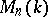, i.e. a mapping assigning to each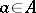a square matrix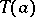of ordersuch that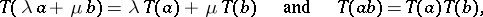(*)

where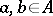,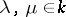. It is usually also required that the unit incorresponds to the identity matrix; sometimesis also required to be finite-dimensional.

Every indecomposable representation of a finite-dimensional semi-simple algebra is equivalent to a direct summand of the regular representation. Hence, every finite-dimensional semi-simple algebra is an algebra of finite (representation) type, i.e. has a finite number of non-isomorphic indecomposable representations. Non-semi-simple algebras can be both of finite and of infinite representation type (e.g. such is the algebra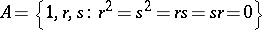). Algebras of infinite type are further divided into algebras of wild type, whose classification problem contains the unsolved problem on matrix pairs (i.e. the problem of simultaneously reducing to canonical form two linear operators on a finite-dimensional space), and algebras of tame type.

Basic problems studied in the representation theory of associative algebras are that of obtaining necessary and sufficient conditions for an algebra to belong to one of the types listed, as well as that of classifying the indecomposable representations in the finite and tame cases. In the general case these problems have not been solved. The description of algebras of finite or tame type and their representations has been obtained for algebras the square of whose radical equals zero (cf. , , ). The Brauer–Thrall problem has been solved, i.e. it has been proved that, over any field, an algebra of infinite type has indecomposable representations of arbitrary high dimension, while over a perfect field there are infinitely many dimensions in each of which there are infinitely many indecomposable representations (cf. , ). Any algebra of finite type over an algebraically closed field has a multiplicative basis, i.e. a basis for which the product of two arbitrary elements in it is either zero or belongs to the basis . The problem of dividing the class of group algebras into tame and wild ones has been completely solved .

Strongly related with representations of associative algebras are representations of other objects: rings, partially ordered sets, lattices, boxes.